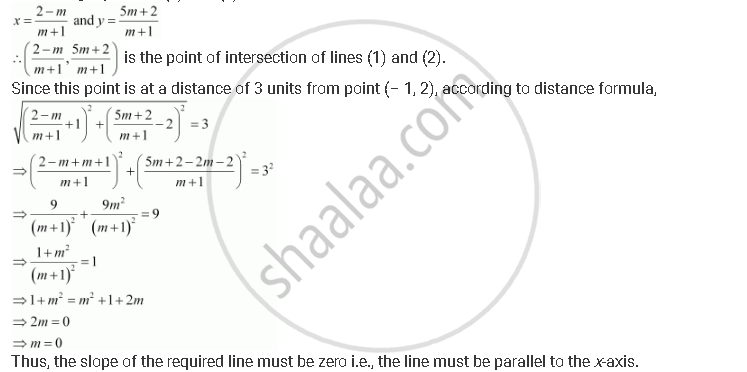CBSE (Arts) Class 11CBSE
Share

# Find the Direction in Which a Straight Line Must Be Drawn Through the Point (–1, 2) So that Its Point of Intersection with the Line X + Y = 4 May Be at a Distance of 3 Units from this Point. - CBSE (Arts) Class 11 - Mathematics

ConceptDistance of a Point from a Line

#### Question

Find the direction in which a straight line must be drawn through the point (–1, 2) so that its point of intersection with the line x + y = 4 may be at a distance of 3 units from this point.

#### Solution

Let y = mx + c be the line through point (–1, 2).

Accordingly, 2 = m (–1) + c.

⇒ 2 = –m + c

⇒ m + 2

∴ mx + m + 2 … (1)

The given line is

x + y = 4 … (2)

On solving equations (1) and (2), we obtainIs there an error in this question or solution?

#### Video TutorialsVIEW ALL 

Solution Find the Direction in Which a Straight Line Must Be Drawn Through the Point (–1, 2) So that Its Point of Intersection with the Line X + Y = 4 May Be at a Distance of 3 Units from this Point. Concept: Distance of a Point from a Line.
S